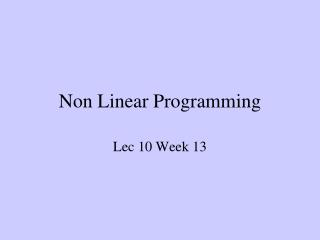DownloadDownload PresentationNon Linear Programming

# Non Linear Programming

Télécharger la présentation## Non Linear Programming

- - - - - - - - - - - - - - - - - - - - - - - - - - - E N D - - - - - - - - - - - - - - - - - - - - - - - - - - -
##### Presentation Transcript

1. Non Linear Programming Lec 10 Week 13

2. NLP • A nonlinear programming problem (NLP) is an optimization problem where the objective function or some of the constraints are nonlinear. Consider the following problem:

3. General Nonlinear Programming Problems objective function constraints

4. Local Minima vs. Global Minima objective function constraints local minimum global minimum

5. Using (b) to eliminate x1 gives: and substituting into (a) :- (c) Optimisation with Equality Constraints Elimination of variables: example:

6. Then using (c): At a stationary point Hence, the stationary point (min) is: (1.071, 1.286)

7. The Lagrange Multiplier Method Consider a two variable problem with a single equality constraint: At a stationary pointwe may write:

8. nontrivial nonunique solutions for dx1 and dx2 will exist. If: This is achieved by setting where  is known as a Lagrange multiplier.

9. If an augmented objective function, called the Lagrangian is defined as: we can solve the constrained optimisation problem by solving:

10. Generalizing: To solve the problem: define the Lagrangian: and the stationary point (points) is obtained from:-

11. Consider the previous example Example again. The Lagrangian is:- Substituting (a) and (b) into (c) gives:

12. which agrees with the previous result.